Next: Jonathan Leech - Noncommutative Up: Universal Algebra and Multiple-Valued Previous: Jennifer Hyndman - Dualizable

## Benoit Larose - Projective graphs and Hedetnyemi's conjecture

 BENOIT LAROSE, Champlain Regional College, Quebec, Canada Projective graphs and Hedetnyemi's conjecture

Let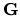be a finite simple graph, which for our purposes we define as a pair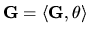where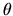is a symmetric and areflexive binary relation on the finite set G. We say thatis n-projective if the only n-ary idempotent operations in the clone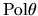are the projections, andis n-quasiprojective if all the n-ary idempotent operations in the clonepreserve every subset of G. We prove that for all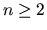, n-projectivity, n-quasiprojectivity and 2-quasiprojectivity are equivalent. (the result also holds for infinite graphs). This can be used to show that complete graphs, odd cycles and Kneser graphs are n-projective for every.

These results are related to Hedetnyemi's conjecture, which states that the chromatic number of the product of two graphs is the minimum of the chromatic numbers of the factors; indeed, this conjecture can be reformulated as follows: every complete graph Kn is multiplicative, i.e. if Kn is a retract of a product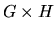then it is a retract of G or H. We show that under certain conditions, a projective graph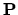is weakly multiplicative, i.e. that ifis a retract of a product of connected graphs then it is a retract of one of the factors. In particular, complete graphs and Kneser graphs are shown to be weakly multiplicative. (Note that there exist Kneser graphs which are not multiplicative (Tardif, Zhou)).

This is joint work with C. Tardif.Next: Jonathan Leech - Noncommutative Up: Universal Algebra and Multiple-Valued Previous: Jennifer Hyndman - Dualizable